# Quadratic Programming Example: Simplex Method

In this section, we provide an example of Quadratic Programming. We will use the simplex method to solve this problem.

##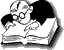Example: Quadratic Programming Problem

Maximize f(x) = 2x1 + 3x2 –x12 – x22

subject to
x1 + x2 ≤ 2
2x1 + x2 ≤ 3

x1, x2 ≥ 0.

Solution.

First, find that a given function is concave or convex.
df(x)/dx1 = 2 – 2x1
df(x)/dx2 = 3 – 2x2
d2f(x)/dx12 = – 2
d2f(x)/dx22 = – 2
d2f(x)/dx1.dx2 = 0

On small screens, use horizontal scrollbar to view full calculation

 H(x) = d2f(x)/dx12 d2f(x)/dx1.dx2 d2f(x)/dx1.dx2 d2f(x)/dx22

 H(x) = – 2 0 0 –2

 I = 1 0 0 1

 λ(I) λ 0 0 λ

 H(x) – λ(I) – 2 0 – λ 0 0 –2 0 λ

 H(x) – λ(I) – 2 – λ 0 0 – 2 – λ

Determinant of H(x) – λ(I) = 0

(– 2 – λ ) X (– 2 – λ ) – 0 X 0 = 0
λ2 + 4λ + 4 = 0
λ2 + 2λ + 2λ + 4 = 0
λ(λ + 2) + 2(λ + 2) = 0
λ = –2

Since λ is negative, the given problem has a concave objective function.

φ (x, λ ) = 2x1 + 3x2 –x12 – x22 + λ1(2 – x1 – x2) + λ2(3 – 2x1 – x2)

Differentiate w.r.t. x1
φ x1 = 2 – 2x1 – λ1 – 2λ2 = – μ1.....(i)

Differentiate w.r.t. x2
φ x2 = 3 – 2x2 – λ1 – λ2 = – μ2.....(ii)

Where μ1 and μ2 are surplus variables.

x1, x2, λ1, λ2, μ1, μ2 ≥ 0
μ1x1 = 0, μ2x2 = 0

Differentiate w.r.t. λ 1
φ λ1 = 2 – x1 – x2 = S1.....(iii)

Differentiate w.r.t. λ 2
φ λ2 = 3 – 2x1 – x2 = S2.....(iv)

Where S1 and S2 are slack variables.

λ1S1 = 0, λ2S2 = 0

### Kuhn Tucker Conditions

From equations (i), (ii), (iii) & (iv), we get

2x1 + λ1 + 2λ2 – μ1 = 2
2x2 + λ1 + λ2 – μ2 = 3
x1 + x2 + S1 = 2
2x1 + x2 + S2 = 3

Introducing artificial variables A1and A2 to the first two equations to obtain a feasible solution of the problem.

Maximize –A1 –A2

subject to

2x1 + λ1 + 2λ2 – μ1 + A1 = 2
2x2 + λ1 + λ2 – μ2 + A2 = 3
x1 + x2 + S1 = 2
2x1 + x2 + S2 = 3

Now, we solve the above problem by Simplex method.

Table 1

Use horizontal scrollbar to view full calculation

cj 0 0 0 0 0 0 0 0 –1 –1
cB Basic variables
B
x1 x2 μ1 μ2 S1 S2 λ1 λ2 A1 A2 Solution values
b (=XB)
–1 A1 2 0 –1 0 0 0 1 2 1 0 2
–1 A2 0 2 0 –1 0 0 1 1 0 1 3
0 S1 1 1 0 0 1 0 0 0 0 0 2
0 S2 2 1 0 0 0 1 0 0 0 0 3
zj–cj   –2 –2 1 1 0 0 –2 –3 0 0

If we ensure that the pairs ( λi, Si) and (μj, xj) are not basic variables simultaneously then the conditions Siλi = 0 and xjμj = 0 will be automatically satisfied.
Although zj – cj is lowest corresponding to λ2 column, it can’t be made a basic variable as S2 is already a basic variable. Hence, A1 departs and x1 enters.

Table 2

cj 0 0 0 0 0 0 0 0 –1
cB Basic variables
B
x1 x2 μ1 μ2 S1 S2 λ1 λ2 A2 Solution values
b (=XB)
0 x1 1 0 –1/2 0 0 0 1/2 1 0 1
–1 A2 0 2 0 –1 0 0 1 1 1 3
0 S1 0 1 1/2 0 1 0 –1/2 –1 0 1
0 S2 0 1 1 0 0 1 –1 –2 0 1
zj–cj   0 –2 0 1 0 0 –1 –1 0

Table 3

cj 0 0 0 0 0 0 0 0 –1
cB Basic Variables
B
x1 x2 μ1 μ2 S1 S2 λ1 λ2 A2 Solution values
b (=XB)
0 x1 1 0 –1/2 0 0 0 1/2 1 0 1
–1 A2 0 0 –1 –1 –2 0 2 3 1 1
0 x2 0 1 1/2 0 1 0 –1/2 –1 0 1
0 S2 0 0 1/2 0 –1 1 –1/2 –1 0 0
zj–cj   0 0 1 1 2 0 –2 –3 0

Since S2 is a basic variable, λ2 can’t be made a basic variable.
Therefore, the variable A2 departs and λ1 enters.

### Final Table: Quadratic Simplex Method

cj 0 0 0 0 0 0 0 0
cB Basic variables
B
x1 x2 μ1 μ2 S1 S2 λ1 λ2 Solution values
b (=XB)
0 x1 1 0 –1/4 1/4 1/2 0 0 1/4 3/4
0 λ1 0 0 –1/2 –1/2 –1 0 1 3/2 1/2
0 x2 0 1 1/4 –1/4 1/2 0 0 –1/4 5/4
0 S2 0 0 1/4 –1/4 –3/2 1 0 –1/4 1/4
zj–cj   0 0 0 0 0 0 0 0

The values for x1 and x2 are 3/4 and 5/4 respectively.
The associated optimal value of the objective function is f(x) = 2 X 3/4 + 3 X 5/4 – (3/4)2 – (5/4)2 = 25/8

•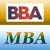MBA & BBA
•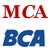BCA & MCA
•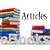Ebooks & Articles
•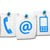Contact us